# Consider these two entries from a fictional table of standard reduction potentials?

Consider these two entries from a fictional table of standard reduction potentials.

X2+ + 2e- => X(s) E=1.70 V
Y2+ + 2e- => Y(s) E=0.14 V

Which pair of species would react under standard conditions?

_X2 and Y
_X2 and Y2
_X and Y
_X and Y2

Concepts and reason
Gibbs free energy of the overall reaction is negative, then the reaction is said to be spontaneous.
From the two half-cell reactions, we can find standard cell potential.
The relation between standard cell potential and Gibbs free energy is as follows: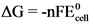From the relation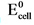is positive then Gibbs free energy is negative. Then that reaction is spontaneous.
And, from the relationis negative then Gibbs free energy is positive. Then that reaction is non-spontaneous.
Reactive species which in a galvanic cell, the oxidation reaction takes place at anode and the reduction reaction takes place at cathode. The standard potential () can be calculated using the following formula: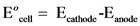Fundamentals
In the two given half cells, one of the half-cell reaction undergo oxidation and other half-cell reaction is reduction.
The reaction which is having, more positive standard reduction potential that reaction undergo reduction and other reaction which is having less positive reduction potential undergo oxidation reaction.
In galvanic cell oxidation reaction takes place at anode and reduction reaction takes place at cathode.

The standard potential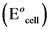can be calculated using the following formula:The relation between standard cell potential and Gibbs free energy is as follows: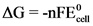The standard reduction potentials for the two half-reactions are as follows: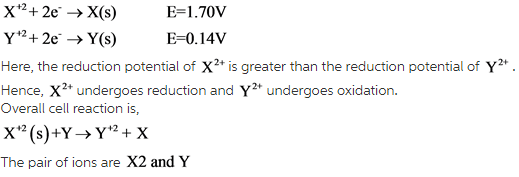Explanation:
The ion which has higher reduction potential undergoes reduction and the other ion or atom undergoes oxidation.

The oxidation half-reaction is as follows:
At anode: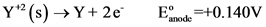The reduction half-reaction is as follows:
At cathode: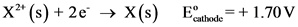Now, calculate the standard potential for this galvanic cell as follows: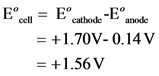Therefore, the value of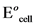is + 1.56 V.
The relation between standard cell potential and Gibbs free energy is as follows:From the relation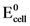is positive then Gibbs free energy is negative. Then that reaction is spontaneous.
Therefore, the pair of species would react under standard conditions are species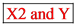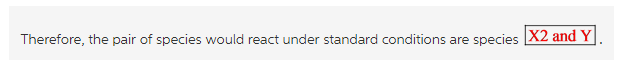Explanation:
The species undergo reaction at standard conditions, the standard cell potential valuewill be positive. Then the corresponding species have negative Gibbs free energy.